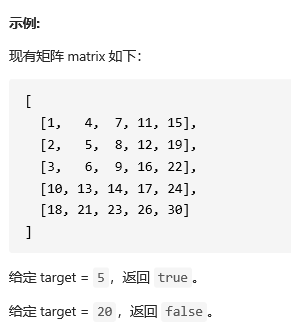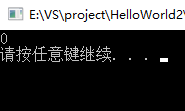• //vector二维数组处理不定长度的问题 for(int i=0;i;i++){ vectorr; string s; getline(cin,s); int k=0; for(int j=0;j>n; for(int i=0;i>a>>b>>c>>d>>e; if(a>v.size()||b>v.size()||c>v.size()||d>v....
1052 卖个萌 (20分)
萌萌哒表情符号通常由“手”、“眼”、“口”三个主要部分组成。简单起见，我们假设一个表情符号是按下列格式输出的：
[左手]([左眼][口][右眼])[右手]
现给出可选用的符号集合，请你按用户的要求输出表情。
输入格式：
输入首先在前三行顺序对应给出手、眼、口的可选符号集。每个符号括在一对方括号 []内。题目保证每个集合都至少有一个符号，并不超过 10 个符号；每个符号包含 1 到 4 个非空字符。
之后一行给出一个正整数 K，为用户请求的个数。随后 K 行，每行给出一个用户的符号选择，顺序为左手、左眼、口、右眼、右手——这里只给出符号在相应集合中的序号（从 1 开始），数字间以空格分隔。
输出格式：
对每个用户请求，在一行中输出生成的表情。若用户选择的序号不存在，则输出 Are you kidding me? @\/@。
输入样例：
[╮][╭][o][~\][/~]  [<][>]
[╯][╰][^][-][=][>][<][@][⊙]
[Д][▽][_][ε][^]  ...
4
1 1 2 2 2
6 8 1 5 5
3 3 4 3 3
2 10 3 9 3
输出样例：
╮(╯▽╰)╭
<(@Д=)/~
o(^ε^)o
Are you kidding me? @\/@
开始没有明白题意。。。
三行存储的分别是左右手，左右眼，口，只不过每行的长度不确定，利用veCTOR二位数组
其中还有很多小细节，，，好好看代码，，
#include<bits/stdc++.h>
using namespace std;
int main(){
//三行表情给出的分别是左右手，左右眼，嘴
vector<vector<string> >v;//vector二维数组处理不定长度的问题
for(int i=0;i<3;i++){
vector<string>r;
string s;
getline(cin,s);
int k=0;
for(int j=0;j<s.length();j++){
if(s[j]=='['){
while(k++<s.length()){
if(s[k]==']')
{
r.push_back(s.substr(j+1,k-1-j));//substr函数是从j+1这个下标开始，截取k-1-j元素
break;
}
}
}
}
v.push_back(r);
}
int n;
cin>>n;
for(int i=0;i<n;i++){
int a,b,c,d,e;
cin>>a>>b>>c>>d>>e;
if(a>v.size()||b>v.size()||c>v.size()||d>v.size()||e>v.size()||a<1||b<1||c<1||d<1||e<1)
cout<<"Are you kidding me? @\\/@"<<endl;//输出\要加转义字符\

else
cout << v[a-1] << "(" << v[b-1] << v[c-1] << v[d-1] << ")" << v[e-1] << endl;//用户是从下标为1开始的
}

return 0;
}


展开全文c++
• vector<vector<int> >num = { { 1，2，3，4 }, { 2，3，4，5}, { 4，5，6，7 }, { 6，7，8，9 } }; vector<vector<int> >nums(n,vector<int>(m,0)); //n行m列
vector<vector<int> >num =
{ { 1，2，3，4 }, { 2，3，4，5}, { 4，5，6，7 },  { 6，7，8，9 } };

vector<vector<int> >nums(n,vector<int>(m,0)); //n行m列 初值为0



展开全文算法
• 在一个 n * m的二维数组中，每一行都按照从左到右递增的顺序排序，每一列都按照从上到下递增的顺序排序。请完成一个高效的函数，输入这样的一个二维数组和一个整数，判断数组中是否含有该整数。 一、解题思路 从...


文章目录
前言一、解题思路二、错误1.错误示范2.错误分析3.错误改正
总结

前言
近期在刷LeetCode上一道题时，遇到了一点点问题，做个小笔记。 剑指 Offer 04. 二维数组中的查找：

在一个 n * m的二维数组中，每一行都按照从左到右递增的顺序排序，每一列都按照从上到下递增的顺序排序。请完成一个高效的函数，输入这样的一个二维数组和一个整数，判断数组中是否含有该整数。一、解题思路
从左下角开始走，利用这个顺序关系可以在O(m+n)的复杂度下解决这个题：
如果当前位置元素比target大，则row– 如果当前位置元素比target小，则col++ 如果相等，返回true 如果越界了还没找到，说明不存在，返回false
#include<iostream>
#include<vector>
using namespace std;

class Solution {
public:
bool findNumberIn2DArray(vector<vector<int>>& matrix, int target) {
int i = matrix.size() - 1, j = 0;
while (i >= 0 && j < matrix.size())
{
if (matrix[i][j] > target)
i--;
else if (matrix[i][j] < target)
j++;
else return true;
}
return false;
}
};

int main() {

Solution s;
vector<vector<int>> matrix{  };
int target = 5;
bool ans = s.findNumberIn2DArray(matrix, target);
cout << boolalpha << ans << endl;
system("pause");
}


二、错误
1.错误示范
一开始我是从右下角开始遍历的，思路同样也没有问题，但是败在细节。
从右上角开始走，利用这个顺序关系可以在O(m+n)的复杂度下解决这个题： 如果当前位置元素比target小，则row++ 如果当前位置元素比target大，则col– 如果相等，返回true 如果越界了还没找到，说明不存在，返回false
错误代码如下（示例）：
class Solution {
public:
bool findNumberIn2DArray(vector<vector<int>>& matrix, int target) {

int r = 0;
int c = matrix.size() - 1 ;

while(r < matrix.size() && c >= 0){
if(matrix[r][c] > target)
c--;
else if(matrix[r][c] < target)
r++;
else
return true;
}
return false;
}
};

乍一看代码跟第一部分从左下角开始遍历没有区别，但是编译总是出现错误：

Line 1033: Char 9: runtime error: reference binding to null pointer of type ‘std::vector<int, std::allocator>’ (stl_vector.h) SUMMARY: UndefinedBehaviorSanitizer: undefined-behavior /usr/bin/…/lib/gcc/x86_64-linux-gnu/9/…/…/…/…/include/c++/9/bits/stl_vector.h:1043:9

2.错误分析
仔细分析，原来是因为vector容器size()的使用出现了问题： LeetCode的测试中还包括二维数组为空[ ] ，在此时matrix.size()会显示为0，而matrix.size()就会报错！！！
代码如下（示例）：
#include<iostream>
#include<vector>
using namespace std;

int main() {
vector<vector<int>>arr{};
int a = arr.size();//注意
cout << a << endl;
system("pause");
}运行正常，结果为0.

该处使用的为arr.size()。
#include<iostream>
#include<vector>
using namespace std;

int main() {
vector<vector<int>>arr{};
int a = arr.size(); //注意
cout << a << endl;
system("pause");
}会报错vector容器超出范围！！！

该处使用的为arr.size()，但是vector为{{ }}！！！
int main() {
vector<vector<int>>arr{ { } };// ！！！
int a = arr.size();
cout << a << endl;
system("pause");
}结果为0，不会报错。
而在第一部分的代码中：
class Solution {
public:
bool findNumberIn2DArray(vector<vector<int>>& matrix, int target) {
int i = matrix.size() - 1, j = 0;
while (i >= 0 && j < matrix.size())
{
if (matrix[i][j] > target)
i--;
else if (matrix[i][j] < target)
j++;
else return true;
}
return false;
}
};

实际上并没有进行到while循环中判断 j < matrix.size()这一步，因为如数组为空，i = -1，不满足i >= 0的条件，实际上在此种条件下没有进入while循环。

3.错误改正
从右下角开始遍历时，加上判断vector二维数组是否为空的条件。
代码如下（示例）：
class Solution {
public:
bool findNumberIn2DArray(vector<vector<int>>& matrix, int target) {

if(matrix.size() < 1 || matrix.size() < 1 )
return false;

int r = 0;
int c = matrix.size() - 1 ;

while(r < matrix.size() && c >= 0){
if(matrix[r][c] > target)
c--;
else if(matrix[r][c] < target)
r++;
else
return true;
}
return false;
}
};


总结
1.注意二维数组中vector为{ }以及{ { } }的情况。其中
vector为{ }，matrix.size()报错。vector为{ { } }，matrix.size()为0。
一般情况下，这两种情况LeetCode都会测试。
2.即使vector为{ }，但是matrix.size()用在if( )条件判断中还是可以用的。
展开全文• vector二维数组注意使用前要分配空间，不然就会出现以下错误： error: reference binding to null pointer of type 'value_type' 错误不唯一 所以最好在vector二维数组定义时就分配好空间 vector<vector<int&...
vector二维数组注意使用前要分配空间，不然就会出现以下错误：
error: reference binding to null pointer of type 'value_type'

错误不唯一 所以最好在vector二维数组定义时就分配好空间
vector<vector<int>> vec(m, vector<int>(n, 0));//初始化一个m行n列的元素值全为0的二维数组

还有值得注意的是在刷题过程中发现，对于vector使用push_back()的耗时要比直接赋值（list[i][j] = a）短得多，比emplace_back耗时也要短（不过这只是暂时碰到的情况，有没有例外不清楚，具体原因也不清楚，等大牛科普一下）反正先用着push_back()就行了
参考资料： https://www.cnblogs.com/ranzhong/p/14330226.html https://blog.csdn.net/wang13342322203/article/details/94323165
展开全文c++
• 在完成CodeForce 234E的时候,想到可以用Vector来完成模拟,下面给出代码 #include<bits/stdc++.h> using namespace std; struct Team{ char name; int score; } team; bool cmp(Team A,Team B) { ...c++ 算法 stl
• 在刷LeetCode的时候需要初始化创建一个行大小为r、列大小为c的vector二维数组，具体的实现方法如下： vector<vector<int>> num(r, vector<int>(c)); 初始化创建r个类型为vector<int>，大小...c++ leetcode 开发语言
• 给定一个 n × n 的矩阵，求它顺时针旋转 90 度的结果，且必须在原矩阵上修改（in-place）。...vector> using namespace std; class Matrix { public:Matrix(); ~Matrix(); void rotate(vector<vectoc++
• 遍历vector二维数组的方法 下标遍历 vector<vector<int>> vec(5, vector<int>(6, 0)); int i,j; for (i = 0; i < vec.size(); i++) { for(j = 0; j < vec[i].size(); j++) cout <<...c++ stl
• 关于vector二维数组作函数参数的问题 vector二维数组有两种定义方式。 两种方式分别对应两种传参形式。 如果使用vector嵌套方式定义，第一维只能使用->调用vector内部函数。 此外vector二维数组还有个注意点： ...c++
• C++获取vector二维数组的行数和列数 代码： #include <iostream> #include <string> using namespace std; int main(){ //创建4*5二维数组，并将数组元素的值都设为1 vector<vector<int>>...c++
• 1.sort()函数，默认的是对二维数组按照第一列的大小对每行的数组进行排序。默认从小到大 #include <iostream> #include<vector> #include<algorithm> using namespace std; int main() { vector...
• 对于动态二维数组a如果不事先定义她的大小，只能放的元素是一个动态数组，a.push_back(vector<int>b),而不能放入一个int类型，a[i].push_back(1),不可行，除非是 vector<vector<int>>a（3）事先定义她的大小了，是...
• vector> using namespace std; int main() { int row, column; cin >> row >> column; //key code:申请空间 vector<vector<int> > a(row, vector<int>(column)); //使用...c++ 数据结构 经验分享
• 之前我一直以为vector不能用vec = {1,2,3}这种方式直接定义，直到我看到下面的代码 vector<vector<int> >num = { { 1, 1, 1, 0, 1, 1 }, { 1, 0, 1, 1, 1, 1 }, { 1, 1, 1, 0, 0, 1 }, { 1, 0, 1, 0,...
• //定义了行数为3列数不定的二维数组 array.size()//返回二维数组的行数 array.size()//返回二维数组第一行的列数 定义一个行列固定的二维vector数组 例： vector<vector<int> > a; a....
• vector 二维数组的传值问题，通过类指针如何实现？ 请问有没有大神指导一下，出血菜鸟一直崩溃。。。c++
• 1. vector二维数组初始化方法: 2.示例代码 unsigned int rows = costMatrix.rows(); unsigned int cols = costMatrix.cols(); vector< vector<double> > costFunc(rows,vector<double>...
• vector二维数组的内存分析 创建一个2x4的二维数组。想到的办法是：先创建一个容器的容器，外层大小的2（2行），然后里面容器小大为4（4列）。 int row=2,col=4; vector<vector<int> > v(row);//v为...
• 原文：... 在LeetCode刷算法的时候发现，使用vector<vector<int>> 时如果声明时不初始化，会报空指针引用错误：reference binding to null pointer of type 'value_type'。 根据《...
• vector构成的二维数组 创建二维数组赋值 遍历二维数组 输出图示： 题目： 在一个二维数组中，每一行都按照从左到右递增的顺序排序，每一列都按照从上到下递增的顺序排序。请完成一个函数，输入这样的一个...剑指offer
• vector&gt; #include &lt;algorithm&gt; using namespace std; bool compare(const vector&lt;int&gt;&amp; a,const vector&lt;int&gt;&amp; b){  if(a &lt; b){  ...
• ## vector二维数组使用

千次阅读 2017-08-31 15:46:32
本文综述了二维数组使用，代码可以跑一下看看效果。 #include #include using namespace std; //method 1 /* int main() { int row, column; cin >> row >> column; //申请空间 vector > a; a.resize(row, ...
• #pragma warning(disable:4996) ...vector> #include <stdio.h> #include <stdlib.h> int main() { double simulationTime = 100; std::vector<std::vector<double>> rec...
• 写一个bool类型的comp函数，比如下面根据第个元素排序： bool cmp1(const vector<int> &a, const vector<int> &b){ return a > b; } sort(allvec.begin(), allvec.end(), cmp1...
• 在一个二维数组中（每个一维数组的长度相同），每一行都按照从左到右递增的顺序排序，每一列都按照从上到下递增的顺序排序。请完成一个函数，输入这样的一个二维数组和一个整数，判断数组中是否含有该整数。 class ......